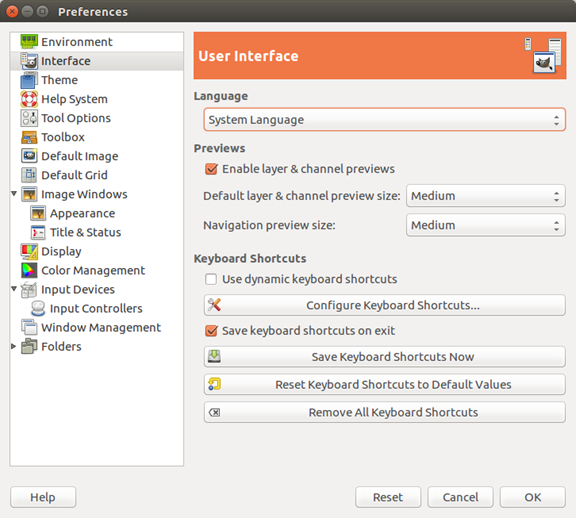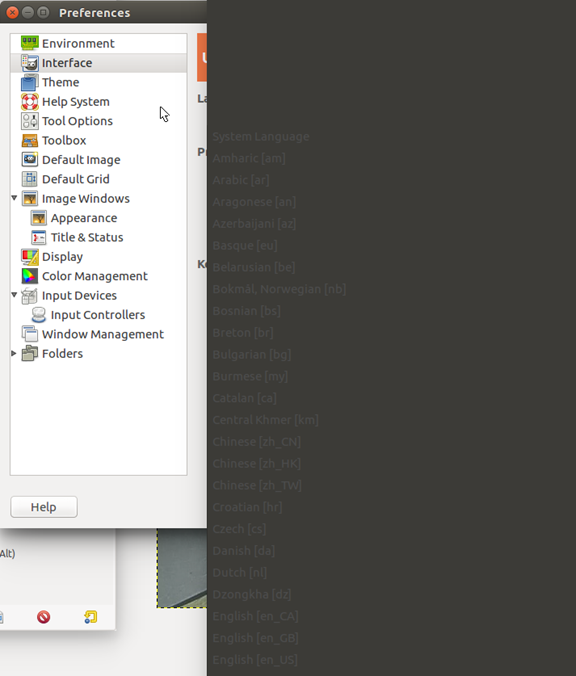# 图像处理 流程

## MATLAB一些简单的图像处理程序

2016-10-10 18:18:48 yangkunpengD 阅读数 9127
• ###### 实现自己的Prisma（基于QT和OpenCV实现Android平台上基于AI的图像处理APP)

本课主要内容是基于QT和OpenCV实现... 重点为QT程序设计、OpenCV.DNN调用深度学习网络和编写完整的Android图像处理程序。 主要基于C++语言，基于QT/QML、OpenCV、torch等基础库和QTcreator。 </div>

28课时 337分钟 301人学习 禾路
免费试看

1

```I=imread('pout.tif');
figure,imshow(I);
[m,n]=size(I);
Inew=zeros(floor(m/2),n);
for i=1:m
for j=1:n
Inew(i,j)=I(2*i-1,j);
end
end
figure,imshow(uint8(Inew))```

2

```clc
clear
x=0:255;
y1=x;
figure,
plot(x,y1,'r')
hold on
y2=255:-1:0;
plot(x,y2,'b')
ylog=255*(log(x+1)/log(256));
plot(x,ylog,'g+')
yexp=255*x.^3/255^3;
plot(x,yexp,'y+')
c=255/255^3;
ynthroot=nthroot((x/c),3);
plot(x,ynthroot,'yo')```
3

```I=imread('moon.tif');
I=double(I);
[m,n]=size(I);
for i=1:m
for j=1:n
if I(i,j)<100
Inew(i,j)=3*I(i,j);
elseif I(i,j)>200
Inew(i,j)=0.8*I(i,j);
else
Inew(i,j)=I(i,j);
end
end
end
figure,imshow(Inew,[])```
4

```clear
clc
I=im2double(I);
[m,n]=size(I);
for i=1:m
for j=1:n
Inew(i,j)=I(i,j)^0.2;
end
end
figure,imshow(I,[])
figure,imshow(Inew,[])```
5

```clear
clc
I=im2double(I);
[m,n]=size(I);
%循环实现
tic
for i=1:m
for j=1:n
Inew(i,j)=255*log(I(i,j)+1)/log(256);
end
end
toc
%查找表
tic
x=0:255;
c=255/log(256);
y=c*log(x+1);
I_log=uint8(y(x+1));
figure,plot(x,y)
toc
figure,imshow(I_log,[])
figure,imshow(I,[])
figure,imshow(Inew,[])```

6

```I=imread('pout.tif');
figure,imshow(I)
[m,n]=size(I);
h=zeros(1,256);
for k=0:255
for i=1:m
for j=1:n
if(I(i,j)==k)
h(k+1)=h(k+1)+1;
end
end
end
end
figure,bar(h)```

```I=imread('pout.tif');
figure,imshow(I)
[m,n]=size(I);
h=zeros(1,256);
for i=1:m
for j=1:n
h(I(i,j)+1)=h(I(i,j)+1)+1;
end
end
figure,bar(h)```

```I=imread('pout.tif');
figure,imshow(I)
[m,n]=size(I);
h=zeros(1,256);
for i=1:m
for j=1:n
h(I(i,j)+1)=h(I(i,j)+1)+1;
end
end
figure,bar(h)
p=h/(m*n);
sum=0;
for i=1:256
sum=sum+p(i);
y(i)=round(sum*255);
end
for i=1:m
for j=1:n
Inew(i,j)=y(I(i,j)+1);
end
end

figure,imshow(unit8(Inew))```

7 邻域处理

```I=imread('pout.tif');
I=double(I);
%卷积模板的不同处理效果不同
%M=ones(3,3)/9;将一幅图模糊处理； M=[0 -1 0;-1 5 -1;0 -1 0];将一幅图锐化处理，改变中心位置的值有不同效果
%M=[1 1 1;0 0 0;-1 -1 -1];指出原始图像中水平边缘存在位置的图像；M=[1 0 -1;1 0 -1;1 0 -1];指出原始图像中垂直边缘存在位置的图像
M=ones(3,3)/9;
[m,n]=size(I);
Inew=zeros(m,n);
%从2开始到m-1忽略边界
for i=2:m-1
for j=2:n-1
Inew(i,j)=I(i-1,j-1)*M(1,1)+I(i-1,j)*M(1,2)+...
I(i-1,j+1)*M(1,3)+I(i,j-1)*M(2,1)+I(i,j)*M(2,2)+...
I(i,j+1)*M(2,3)+I(i+1,j-1)*M(3,1)+I(i+1,j)*M(3,2)+...
I(i+1,j+1)*M(3,3);
end
end
figure,imshow(uint8(Inew))```

## 遥感图像处理流程

2013-04-03 19:05:58 liminlu0314 阅读数 19165
• ###### 实现自己的Prisma（基于QT和OpenCV实现Android平台上基于AI的图像处理APP)

本课主要内容是基于QT和OpenCV实现... 重点为QT程序设计、OpenCV.DNN调用深度学习网络和编写完整的Android图像处理程序。 主要基于C++语言，基于QT/QML、OpenCV、torch等基础库和QTcreator。 </div>

28课时 337分钟 301人学习 禾路
免费试看

1．降噪处理

（1）除周期性噪声和尖锐性噪声图1 消除噪声前图2 消除噪声后

（2）除坏线和条带图3 去条纹前图4 去条纹后图5 去条带前图6 去条带后

2．薄云处理

3．阴影处理

1．图像配准

（1）影像对栅格图像的配准图7 图像配准前图8 图像配准后

（2）影像对矢量图形的配准

2．几何粗纠正

3．几何精纠正

（1）图像对图像的纠正

（2）图像对地图（栅格或矢量）图9 参考地形图图10 待纠正影像（3）图像对已知坐标点（地面控制点）

4．正射纠正1．彩色合成图13真彩色合成( TM321)图14 假彩色合成(TM432)

2．直方图变换图15 直方图拉伸前（原图偏暗）图16 直方图拉伸后图17 直方图拉伸前（原图对比度不强）图18 直方图拉伸后（线性拉伸）

3．密度分割图19 原始图像图20 密度分割图像

4．灰度颠倒图21 灰度颠倒前图22 灰度颠倒后

5．图像间运算图23 原始图像图24 NDVI植被指数图像

6．邻域增强图25 原始图像图26 拉普拉斯滤波图像（5×5）

7．主成分分析图27 第一主成分图28 第二主成分图29 第三主成分图30 第四主成分图31第五主成分图32 第六主成分

8．K-T变换图33 第一主分量（亮度）图34 第二主分量（绿度）图35第三主分量

9．图像融合图36 多光谱影像图 37高分辨率影像1．按ROI裁剪2．按文件裁剪

3．按地图裁剪1．图像镶嵌图42镶嵌左影像图43 镶嵌右影像2．影像匀色图45 匀色前影像图46 匀色后影像

1．目视判读2．图像分类

（1）监督分类图48 原图像图49 监督分类图像

（2）非监督分类

（3）其他分类方

## 图像处理小白之图像处理一般步骤

2019-03-11 16:38:29 qq_35594560 阅读数 1861
• ###### 实现自己的Prisma（基于QT和OpenCV实现Android平台上基于AI的图像处理APP)

本课主要内容是基于QT和OpenCV实现... 重点为QT程序设计、OpenCV.DNN调用深度学习网络和编写完整的Android图像处理程序。 主要基于C++语言，基于QT/QML、OpenCV、torch等基础库和QTcreator。 </div>

28课时 337分钟 301人学习 禾路
免费试看

## GIMP - GNU 图像处理程序 - 中文版

2018-06-14 22:11:00 chengyq116 阅读数 1615
• ###### 实现自己的Prisma（基于QT和OpenCV实现Android平台上基于AI的图像处理APP)

本课主要内容是基于QT和OpenCV实现... 重点为QT程序设计、OpenCV.DNN调用深度学习网络和编写完整的Android图像处理程序。 主要基于C++语言，基于QT/QML、OpenCV、torch等基础库和QTcreator。 </div>

28课时 337分钟 301人学习 禾路
免费试看

## GIMP - GNU 图像处理程序 - 中文版

1. Edit -> Preferences -> Interface2. Chinese [zh_CN]3. 重启 GIMP 即可。

## FPGA图像处理之路，从此开始

2017-09-02 22:35:31 qq_39210023 阅读数 23820
• ###### 实现自己的Prisma（基于QT和OpenCV实现Android平台上基于AI的图像处理APP)

本课主要内容是基于QT和OpenCV实现... 重点为QT程序设计、OpenCV.DNN调用深度学习网络和编写完整的Android图像处理程序。 主要基于C++语言，基于QT/QML、OpenCV、torch等基础库和QTcreator。 </div>

28课时 337分钟 301人学习 禾路
免费试看

FPGA图像处理之路，从此开始，接下来，让我们把时间交给“图像处理”。一休哥在动笔之前，一直在犹豫，反复思考着一个问题，这个问题一直困扰着我，“FPGA在图像处理领域中的地位？”DDR2、DDR3、Zynq、PCIE、USB3.0、千兆网、LVDS、CORDIC，这些新技术的出现为我们提供了新的思路与解决方案，让FPGA拥有更大的潜能，FPGA将会在图像处理领域有更加卓越的表现。